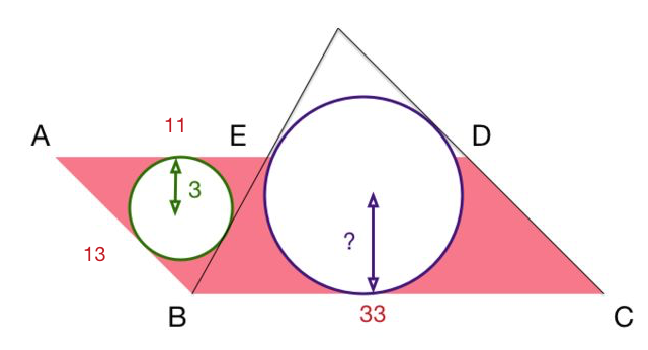# Hideous Arrangement of Circles

Geometry Level 4$ABCD$ is a parallelogram where $AB=13$ and $BC=33$. A point $E$ is taken on $AD$ such that $AE=11$. If the inradius of $\triangle ABE$ is of length $3$, find the radius of the circle touching $EB$, $DC$ and $BC$.

×